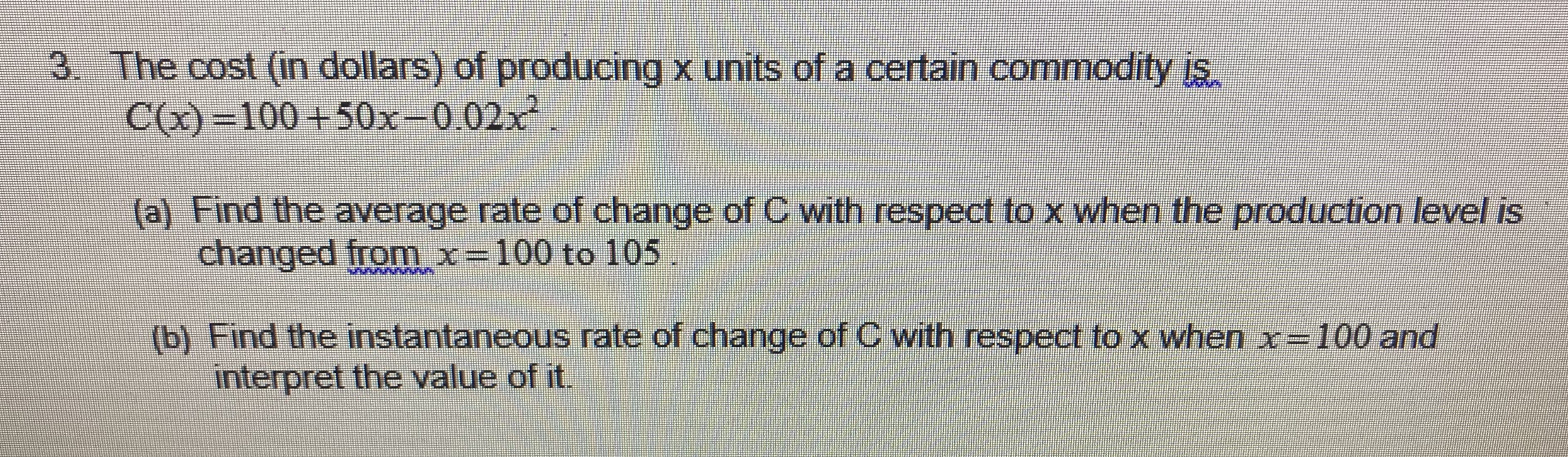3. The cost (in dollars) of producing x units of a certain commodity is.C()-100+50x-0.02x2(a) Find the average rate of change of C with respect to x when the production level ischanged from x- 100 to 105b) Find the instantaneous rate of change of C with respect to x when x- 100 andinterpret the value of it.

Questionhelp_outlineImage Transcriptionclose3. The cost (in dollars) of producing x units of a certain commodity is. C()-100+50x-0.02x2 (a) Find the average rate of change of C with respect to x when the production level is changed from x- 100 to 105 b) Find the instantaneous rate of change of C with respect to x when x- 100 and interpret the value of it. fullscreen
Step 1

a) To calculate the average rate of change of C(x) from x1 = 100 and x2 = 105.

Step 2

Calculate the average rate of change of C(x) as follows.

Step 3

b) To find the instantaneous rate of change of C with r...

Want to see the full answer?

See Solution

Want to see this answer and more?

Our solutions are written by experts, many with advanced degrees, and available 24/7

See Solution
Tagged in

Calculus